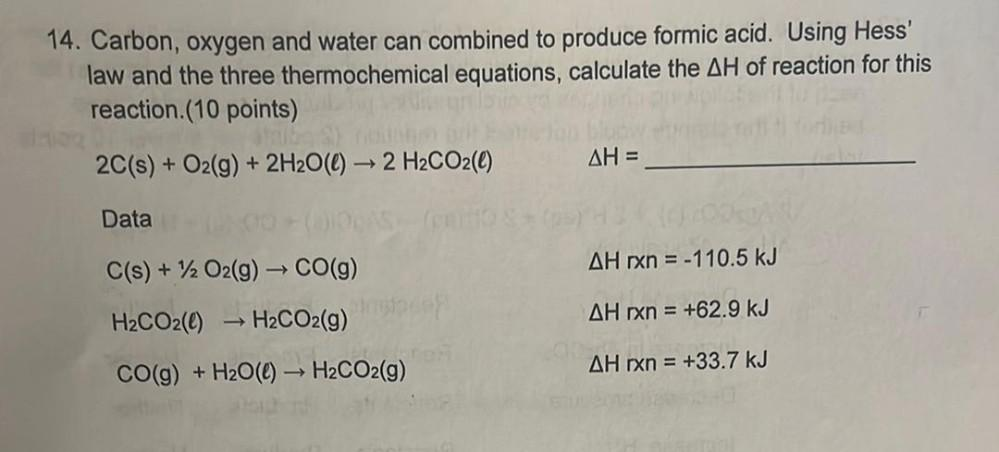Question:

# 14. Carbon, oxygen and water can combined to produce formic acid. Using Hess' law and the three thermochemical equations, calcul14. Carbon, oxygen and water can combined to produce formic acid. Using Hess' law and the three thermochemical equations, calculate the AH of reaction for this reaction (10 points) 2C(s) + O2(g) + 2H2O(l) → 2 H2CO2(e) AH = Data C(s) + 12 O2(g) → CO(9) AH rxn = -110.5 kJ H2CO2(0) H2CO2(g) AH rxn = +62.9 kJ CO(g) + H2O() → H2CO2(g) AH rxn = +33.7 kJ